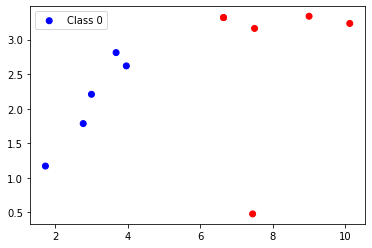# The Algorithm breakdown

• The Gini Index
• The Split
• Building the tree
• Generate Predictions
• Apply to the case study

# The Gini Index

The Gini Index, aslo reffered as the Gini Impurity, is calculated by subtracting the sumation of the probabilities of each class in the dataset

$$Gini = \sum_{i=1}^{C} (p_i) * (1 - p_i)$$

Where $p$ is the probability of each class $C$

Gini index can be discribed as the cost function of the Classification and Regression Trees (CART) at the split node. The Gini Index estimates how good a split is by taking into account how mixed the classes are at the split node. Gini score of $0$ results to a perfect separation where a Gini score of $50/50$ results in an equal distribution of $0.5$ for each class.

#### Gini Example

Supose we have 2 classes of data with 2 rows in class. For a perfect split of $0$ we need the data inth first or the second row to belong either in the first or the secind class.
First we need to calculate the ratio between the classes in each group.

ratio = mumber_of(class) / number_of(rows)

So the Gini Index will be:

gini_index = sum(ratio * (1 - ratio))


For the Gini Index (GI) to have correct output need to introduce the size of each group relave to the entire samples in the parent node

So, the GI will be:

gini_index = sum(ratio * (1 - ratio)) * (size_of_group/total_samples)


import matplotlib.pyplot as plt
import pandas as pd
import altair as alt


def gini_index(groups, classes):
# count the samples at split node
n_instances = float(sum([len(group) for group in groups]))

gini = 0.0
for group in groups:
size = float(len(group))
# prevent zero division
if size == 0:
continue
score = 0.0

# group score based on the score of each class
for class_val in classes:
p = [row[-1] for row in group].count(class_val) / size
score += p * p
# apply the formula
gini += (1.0 - score) * (size / n_instances)

return gini


# test the gini function

groups1 = [
[[1, 1], [1, 0]],
[[1, 1], [1, 0]]
]

groups2 = [
[[1, 0], [1, 0]],
[[1, 1], [1, 1]]
]

classes = [0,1]

print(gini_index(groups1, classes))
print(gini_index(groups2, classes))


0.5
0.0


#### Create the splite node and the dataset

The split node is composed by a value and an attribute in the dataset

Splitting the dataset means to

• loop over the rows in the dataset
• check if the attributes are below or above the split value
• append the values to the left or the right group

def test_split(index, value, dataset):
left, right = list(), list()
for row in dataset:
if row[index] < value:
left.append(row)
else:
right.append(row)
return left, right


# Select the best split point for a dataset
def get_split(dataset):
class_values = list(set(row[-1] for row in dataset))
b_index, b_value, b_score, b_groups = 999, 999, 999, None
for index in range(len(dataset)-1):
for row in dataset:
groups = test_split(index, row[index], dataset)
gini = gini_index(groups, class_values)
if gini < b_score:
b_index, b_value, b_score, b_groups = index, row[index], gini, groups
return {'index':b_index, 'value':b_value, 'groups':b_groups}


dataset = [[2.771244718,1.784783929,0],
[1.728571309,1.169761413,0],
[3.678319846,2.81281357,0],
[3.961043357,2.61995032,0],
[2.999208922,2.209014212,0],
[7.497545867,3.162953546,1],
[9.00220326,3.339047188,1],
[7.444542326,0.476683375,1],
[10.12493903,3.234550982,1],
[6.642287351,3.319983761,1],
[6.642287351,3.319983761,1]]

dataset

0.476683375

x1, x2, y = list(), list(), list()

for x_val1, x_val2, y_val in dataset:
x1.append(x_val1)
x2.append(x_val2)
y.append(y_val)

print(x1)
print("==================")
print(x2)
print("==================")
print(y)

[2.771244718, 1.728571309, 3.678319846, 3.961043357, 2.999208922, 7.497545867, 9.00220326, 7.444542326, 10.12493903, 6.642287351, 6.642287351]
==================
[1.784783929, 1.169761413, 2.81281357, 2.61995032, 2.209014212, 3.162953546, 3.339047188, 0.476683375, 3.234550982, 3.319983761, 3.319983761]
==================
[0, 0, 0, 0, 0, 1, 1, 1, 1, 1, 1]

colors = {0:'blue', 1:'red'}
color_ls = [colors[i] for i in y]

with plt.ion():

plt.scatter(x1, x2, c=color_ls)
plt.legend(['Class 0', 'Class 1'])
plt.axis()
plt.show()class_values = list(set(row[-1] for row in dataset))
class_values

[0, 1]
split = get_split(dataset)
print('Split: [X%d < %.3f]' % ((split['index']+1), split['value']))

Split: [X1 < 6.642]


#### Create the Tree

To build the tree involves the following steps

• Need to have terminal nodes
• Have Recursive splitting
• and finally build the tree
def terminal_node(group):
outcomes = [row[-1] for row in group]
return max(set(outcomes), key=outcomes.count)

def split(node, max_depth, min_size, depth):
left, right = node['groups']
del(node['groups'])
# check for a no split
if not left or not right:
node['left'] = node['right'] = terminal_node(left + right)
return
# check for max depth
if depth >= max_depth:
node['left'], node['right'] = terminal_node(left), terminal_node(right)
return
# process left child
if len(left) <= min_size:
node['left'] = terminal_node(left)
else:
node['left'] = get_split(left)
split(node['left'], max_depth, min_size, depth+1)
# process right child
if len(right) <= min_size:
node['right'] = terminal_node(right)
else:
node['right'] = get_split(right)
split(node['right'], max_depth, min_size, depth+1)

def build_tree(train, max_depth, min_size):
root = get_split(train)
split(root, max_depth, min_size, 1)
return root

def print_tree(node, depth=0):
if isinstance(node, dict):
print('%s[X%d < %.3f]' % ((depth*' ', (node['index']+1), node['value'])))
print_tree(node['left'], depth+1)
print_tree(node['right'], depth+1)
else:
print('%s[%s]' % ((depth*' ', node)))

tree = build_tree(dataset, 1, 1)
tree

{'index': 0, 'value': 6.642287351, 'left': 0, 'right': 1}